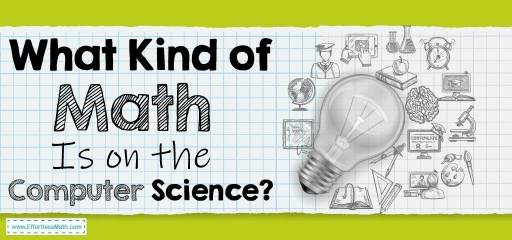# What Kind of Math Is on the Computer Science?Computer Science is one of the fascinating fields for people who have a passion for computer systems. Some of its components are relatively easy to understand. However, some aspects scare many people.

To get higher-level jobs and become a proficient computer scientist, an understanding of math is necessary. This is something that discourages people from taking computer science.

If you’re struggling to decide on the field because of math, you’ve come to the right place. Here is everything you need to know about what kind of math is necessary for computer science.

## Why Is Math Required for Computer Science?

Before diving into the details of math required for this subject, you must know the why behind it. Firstly, some jobs require computer scientists to use math daily. Secondly, math allows students to develop logic that will be necessary when they start working.

## What Kind of Math Is on The Computer Science?

There are many types of math courses that you can take for computer science. This depends on the aspect of the field that you want to excel in. Here are the different kinds of math that you will find in computer science programs:

### 1- Calculus

Calculus is one of the most prevalent branches of math that you’ll find for computer science. The subject involves attempting questions by the use of graphs. Typically, students are expected to know of up to Calculus III.

This type of math is necessary for most professions related to computer science. These include computer graphics, computer security, and much more.

### 2- Logic for Mathematics

It is no secret that programming involves thinking logically to predict a suitable outcome. That is why Logic for Mathematics is an integral part of the field. In this branch, students learn problem-solving skills by theory applications relevant to computers.

Besides that, to enroll in the course of logic, specific requirements need to be fulfilled. For example, some institutes require the student to pass a math test of a basic level.

### 3- Discrete Math

This branch of math involves studying objects that can be quantified. Typically, discrete math forms the foundation of most computer science professions. Whether you want to become a software engineer or a programmer, understanding this subject will be necessary.

By taking this course, you can land any computing job with a computer science degree. That is because it will allow you to understand various programming algorithms.

### 4- Linear Algebra

Linear algebra holds the same importance as discrete math in the field of computer science. That is because its applications in the career paths are vast. Most of the algorithms that computer scientists have to create are coded using this kind of math.

For example, optimization algorithms are implemented using this branch. Besides that, linear algebra is also integral in machine learning jobs.

## Final Words

That was your complete guide to what kind of math is relevant for computer science. You need math even if you just want to be a web developer or a machine learning specialist. The above-mentioned four courses are vital and offered by most degree programs. You can even find online lessons if you are self-studying.

### What people say about "What Kind of Math Is on the Computer Science? - Effortless Math: We Help Students Learn to LOVE Mathematics"?

No one replied yet.

X
30% OFF

Limited time only!

Save Over 30%

SAVE \$5

It was \$16.99 now it is \$11.99